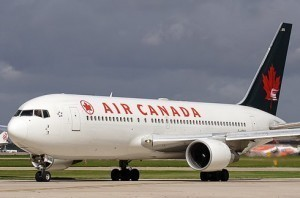•• # What is the size of a Boeing 767?

Like other Boeing airliners, the size of the Boeing 767 will depend on the models.The following are some of the Boeing 767 variants that have been released.

### Dimensions of the Boeing 767 200

The 767 200 has a passenger capacity of 181 for 3 class and 224 for 2 class. For 1 class it is 255. Its cargo capacity is 2,875 sq ft (81.4 m3). The length of the 767 200 is 159 ft 2 in (equal to 48.5 m).

Its wingspan is 156 ft 1 in or 47.6 m. The wing area is 3,050 sq ft (283 m2). Its fuselage height is 17 ft 9 in (5.41 m), with the width being 16 ft 6 in (5.03 m).

The cabin width is 15 ft 6 in (abut 4.72 m). The size of the Boeing 767 gives it an empty operating weight of 176,650 lbs (80,130 kg). The maximum takeoff weight is 315,000 lbs (142,880 kg).

The range of the 767 200 is 3,950 nmi (7,300 km). The cruise speed is Mach 0.80 (530 mph or 861 km/h). This is at 35,000 ft. The maximum cruise speed is 913 km/h or Mach 0.88 or 493 knots.

### Dimensions of the Boeing E 767

The flight crew of the Boeing E 767 is two. Its length is 159 ft 2 in (48.5 m). The 767 has a height of 52 ft (15.8 m) and a wingspan of 156 ft 1 in (47.6 m). The size of the Boeing 767 gives it an empty weight of 188,705 lbs (85,595 kg). The loaded weight of the E 767 is 284,110 lbs (equivalent to 128,870 kg).

The E 767 has a maximum take off weight of 385,000 lbs (175,000 kg). The highest speed possible is Mach 0.85. The cruise speed is Mach 0.80 (equal to 530 mph or 851 km/h).

The range is 5,600 nmi (10,370 km) The E 767’s service ceiling is 40,100 ft (about 12,200 m). It has an endurance of 9.25 hours on station (at 1,000 nautical miles radius). If the nautical mile radius is 300, the endurance is 13 hours.

### Dimensions of the Boeing 767 300

The size of the Boeing 767 allows for a passenger capacity of 218 for three class, 269 for 2 class and 350 for 1 class. Its cargo is good for up to 3,770 sq ft (106.8 m3). The length of the 767 300 is 160 ft 3 in (54.9 m). The wingspan is 156 ft 1 in (47.6 m) with a total wing area of 3,050 sq ft (283 m3).

Its fuselage height and width is similar to the 767 200 (that is 17 ft 9 in and 16 ft 6 in). The cabin width is 15 ft 6 in (4.72 m). Its empty weight is 189,750 lbs (86,070 kg).

The maximum take off weight is 350,000 lbs (158,760 kg) with a maximum range of 3,850 nmi or 7,300 km. The cruise speed is Mach 0.80 or 851 km/h. The maximum cruise speed is Mach 0.88 or 913 km/h.

The size of the Boeing 767 will vary a bit with respect to other variants. However, most other 767 models have the same cabin width and fuselage dimensions.